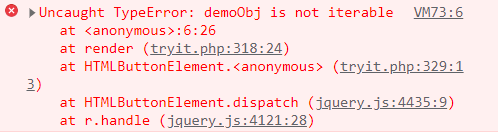Open in App
Not now

• Last Updated : 05 Jan, 2023

The spread syntax is used when expanding an iterable in places where many arguments or elements are expected. It also allows us the privilege to obtain a list of parameters from an array. The spread syntax was introduced in ES6 JavaScript. The spread syntax is different from the rest operator as the spread operator expands any iterable into a list of elements whereas the rest operator is used to add other information supplied.

The spread syntax can be used in three places:

Syntax:

```// spread in function call
function(...obj){ // function body }

Parameter:

• valueToSpread: The iterable that is to be assigned to the new variable is mentioned here. It can be an Array or a String.

Return Type: It returns the argument list of the object passed after three dots.

Spread syntax in Function arguments: The spread syntax can be used in to pass values in a function. It is helpful in situations where we do not know the exact number of arguments that are to be passed. This helps to replace the inbuilt apply() method

Example 1: In this example, we will use the spread syntax in the function arguments

## Javascript

 `function` `multiply(...objects){ ` `    ``var` `ans = 1; ` `    ``for``(``var` `i = 0; i < objects.length; i++){ ` `        ``ans *= objects[i]; ` `    ``} ` `    ``console.log(ans); ` `} ` `multiply(8, 2); ` `multiply(9, 4, 6);`

Output:

```16
216```

Spread syntax in Array literal: The spread syntax is very useful in array literal as it can be used for cloning an array. If we do cloning with inbuilt array methods or assignment operator it can cause some issues as the inbuilt method copy the reference(deep copy) of the array and any changes made in the new array will reflect in the old array.

Example 2: In this example, we will use the spread syntax in Array literal.

## Javascript

 `var` `alpha1 = [``'a'``, ``'b'``, ``'c'``]; ` `var` `alpha2 = [``'d'``, ``'e'``, ``'f'``]; ` ` `  `// Concatenating using three dots ` `alpha1 = [...alpha1, ...alpha2];  ` `console.log(alpha1);`

Output:

`['a', 'b', 'c', 'd', 'e', 'f']`

Spread syntax in object literal: The problem of deep copy also arises in cloning an object using the Assignment operator(‘=’). So, spread syntax helps to overcome this problem and create a new object with shallow copy i.e. changes made in the new object will not reflect in the old object.

Example 3: In this example, we will use the spread syntax in object literal.

## Javascript

 `var` `obj1 = { ` `    ``name: ``'Sham'``, ` `    ``age: 20, ` `    ``skill: ``"Java"` `} ` `var` `obj2 = {...obj1}; ` `console.log(obj2);`

Output:

`{name: 'Sham', age: 20, skill: 'Java'}`

Apart from these examples, we can also use the spread operator to pass arguments in constructors. This is useful when we have data in the format of an array that cannot be directly passed as an array so the spread operator converts it into a list of parameters and the data is accepted.

Example: In this example, we will pass the date as an array and create a new date object.

## Javascript

 `var` `date = [2000, 6, 15]; ` `var` `newDate = ``new` `Date(...date); ` `console.log(newDate);`

Output:

`Sat Jul 15 2000 00:00:00 GMT+0530 (India Standard Time)`

Errors and Exceptions: It must be understood that only those objects can be spread which are iterable. For eg. spreading a plain object will throw an error that the object is not iterable.

Example:

## Javascript

 `const demoObj = {  ` `  ``name: ``'Ram'``, ` `  ``age: ``'22'` `}; ` `const cloneObj = [...demoObj];`

Output:We have a complete list of Javascript ES6 features, to check those please go through this Introduction to ES6 article.

We have a Cheat Sheet on Javascript where we covered all the important topics of Javascript to check those please go through Javascript Cheat Sheet-A Basic guide to JavaScript.

My Personal Notes arrow_drop_up
Related Articles